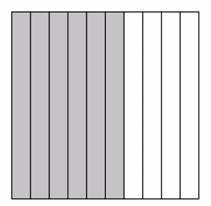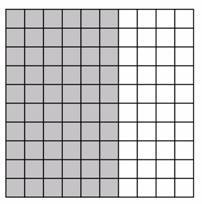# Fraction Equivalence

Alignments to Content Standards: 4.NF.C.5

Explain why $\frac{6}{10} = \frac{60}{100}$. Draw a picture to illustrate your explanation.

## IM Commentary

The explanation below is a teacher-level explanation. Students may not articulate every detail, but the basic idea for a case like the one shown here is that when you have equivalent fractions, you have just cut the pieces that represent the fraction into more but smaller pieces. Explaining fraction equivalences at higher grades can be a bit more involved (e.g. $\frac68 = \frac{9}{12}$), but it can always be framed as subdividing the same quantity in different ways.

Note that there is a subtlety with the idea of "equal pieces." It is easiest to show this with a drawing where the "pieces" are represented by congruent figures; later students will deal with figures that are not congruent but have equal area. The figures shown in the solution show congruent pieces, avoiding this complexity.

## Solution

The picture below shows a square divided into ten equal pieces. The square represents 1. Since six of them are shaded, the shaded part represents $\frac{6}{10}$.If we divide each of the ten pieces into ten smaller, equal-sized pieces, the square is divided into $10 \times 10 = 100$ equal-sized pieces, and each small piece represents $\frac{1}{100}$.The six shaded pieces are now each divided into ten pieces as well, so there are $6 \times 10$ shaded pieces. The shaded area represents $\frac{60}{100}$.

Since the area of the square that is shaded hasn’t changed, the two fractions represent the same amount, so the two fractions are equal.